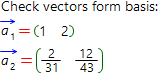# Check vectors form the basis online calculatorThe basis in n-dimensional space is called the ordered system of n linearly independent vectors.

For the following description, intoduce some additional concepts.

Expression of the form:

λ11 + λ22 + ... + λnn

, where λi − some scalars and i=[0; n] is called linear combination of the vectors1 ,2 , ... ,n .

If there are exist the numbers λ1, λ2, ... , λn such as at least one of then is not equal to zero (for example λj0) and the condition:

λ11 + λ22 + ... + λnn = 0

is hold, the the system of vectors1 ,2 , ... ,n − is called linear-dependent.

If the equality above is hold if and only if, all the numbers λ1 = λ2 = ... = λn = 0, then the system of vectors1 ,2 , ... ,n − is called linear-independent.

The basis can only be formed by the linear-independent system of vectors. The conception of linear dependence/independence of the system of vectors are closely related to the conception of matrix rank.

Our online calculator is able to check whether the system of vectors forms the basis with step by step solution for free.

Check vectors form basis1 by:2 by:1 = { }2 = { }